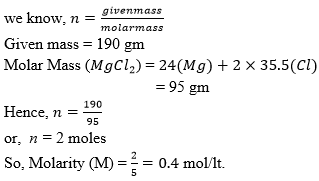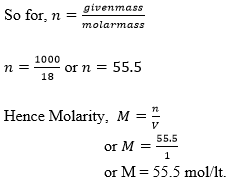# Molarity Formula

## Molarity Formula | Molarity Definition and Formula

The term ‘molarity’ is used to express the concentration of a solute in a solution. There are many such other methods which can be used to convey the percentage composition of any solution, e.g molality, normality, ppm etc. To find the molarity of a binary solution, we simply divide the number of moles of solute by the volume of solution (in litres).
$M = \frac{n}{V}$
where ‘’ is the Molarity, ‘’ is the number of moles of solute and ‘’ is the volume of the solution is litres.
An important point to note here is that the Volume () taken into consideration is of the ‘Solution’ and not the ‘Solvent’. This can be little confusing sometimes so I will give an easy example to understand this much better.

Case I:
Solute taken is solid e.g 1 mole of NaCl
Solvent taken is 2 lt. of water
Now on adding 1 mole of NaCl to 2 lt. of water the volume of the solution is approximately equal to the volume of water taken initially. i.e 2lt. This is because addition of a solid solute does not change the volume of the solvent significantly.
Hence Molarity,
$M = \frac{n}{V}$
or, $M = \frac{1}{2} = 0.5$

Case II:
Solute taken is 200 ml of a solution containing 5 moles of HCl
Solvent is 800 ml water
Now when the solute and solvent are mixed the total volume of the solution will become
200 + 800 = 1000 ml or 1 lt.
The molarity of the solution then can be calculated as,
$M = \frac{5}{1} = 5$

Example: Calculate the molarity of a solution containing 190 gm of MgCl2 in 5 lt. of water.
Solution:
In this example we have been provided with weight of the solute and not the number of moles which we actually require to find the molarity. So the first step would be to find the number of moles of MgCl2 present in 95 gm of the same.Question: Calculate the molarity of 1lt. pure water.
Options:
(a) 1000 M
(b) 18 M
(c) 55.5 M
(d) not sufficient information